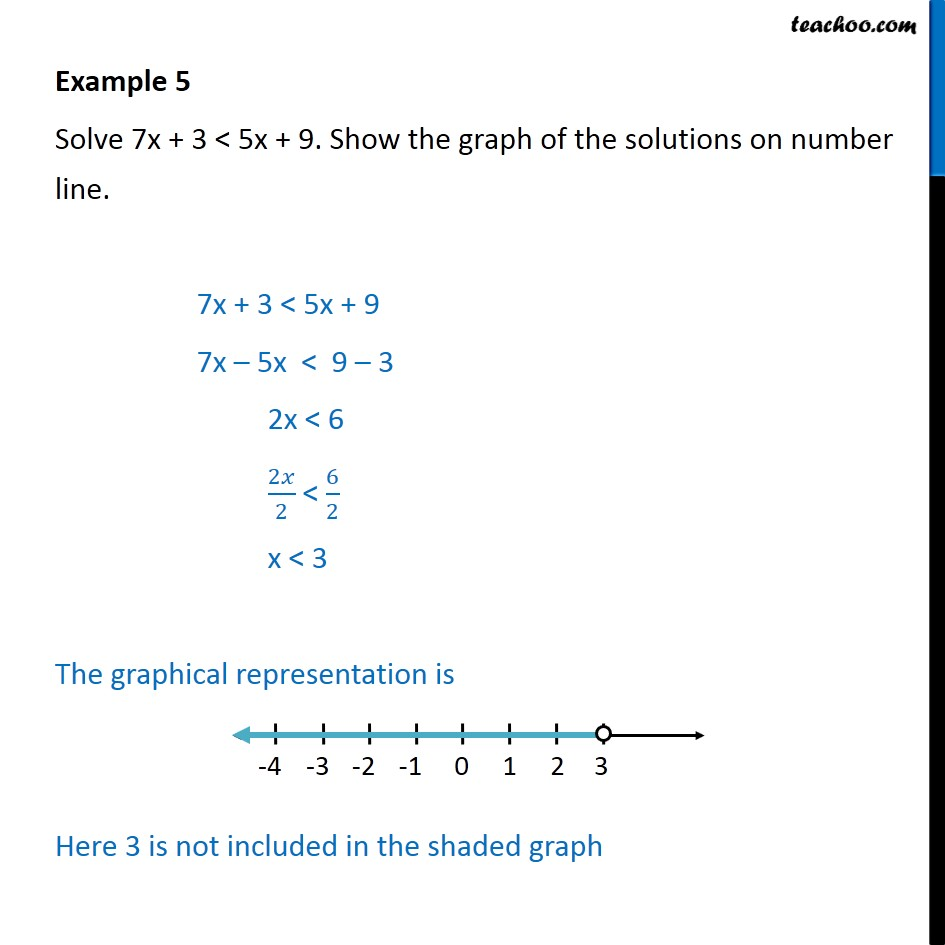1. Chapter 6 Class 11 Linear Inequalities
2. Serial order wise
3. Examples

Transcript

Example 5 Solve 7x + 3 < 5x + 9. Show the graph of the solutions on number line. 7x + 3 < 5x + 9 7x 5x < 9 3 2x < 6 2 2 < 6 2 x < 3 The graphical representation is Here 3 is not included in the shaded graph

Examples